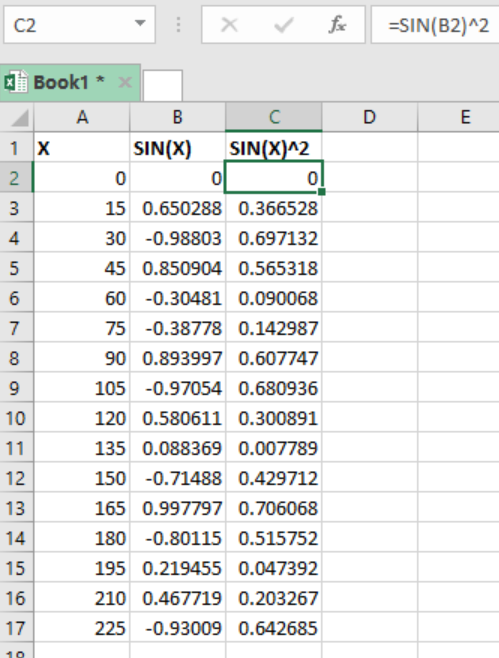Get instant live expert help with Excel or Google Sheets“My Excelchat expert helped me in less than 20 minutes, saving me what would have been 5 hours of work!”

#### Post your problem and you’ll get Expert help in seconds.

Your message must be at least 40 characters
Our professional Expert are available now. Your privacy is guaranteed.

# How to Write and Use Sin Squared in Excel

While working with trigonometric functions, sometimes we are required to use a square of either the sine, cosine or tangent. This can be challenging, especially if you are not aware of how it is written. This article provides a clear guide on how to write and use SIN squared in excel.

## Example

For illustrative purposes, let us consider the following example;Figure 1: Sine squared in excel

In this example, we have the angle, marked as X. we also have the sine of the angle, marled as SIN(X). The sine squared is marked as SIN(X)^2 in column C.

To get the sine squared we simply square the sine. This can be done as shown below;

`=SIN(X)^2`

Note that the results for the sine squared do not have negative. This is because the negatives shall cancel each other during the multiplication to only remain with positives.

## Instant Connection to an Expert through our Excelchat Service

Most of the time, the problem you will need to solve will be more complex than a simple application of a formula or function. If you want to save hours of research and frustration, try our live Excelchat service! Our Excel Experts are available 24/7 to answer any Excel question you may have. We guarantee a connection within 30 seconds and a customized solution within 20 minutes.

Solution examplesIF SUM OF RANGE IS MORE THAN ZERO,THEN SUM IF THE RANGE IS BLANK THEN BLANK IF THE RANGE HAS ONLY ZERO,THEN ZERO HOW SHALL I COMBINE THE ABOVE THREE IN A SINGLE CELL??
Solved by M. D. in 18 minsi need a formula to calculate previous day intraday high from google finance to google sheet. I AM USING THIS ONE =GOOGLEFINANCE(A2,"high",today()-1) BUT ITS NOT WORKING AND GIVING ME ERROR "When evaluating GOOGLEFINANCE, the query for the symbol: 'HDFCBANK' returned no data." HERE A2 IS "HDFCBANK" A TICKER.. AND I ALSO DONT WANT DATE COLUMN WITH THE PREVIOUS DAY INTRADAY HIGH PRICE.
Solved by G. E. in 40 mins